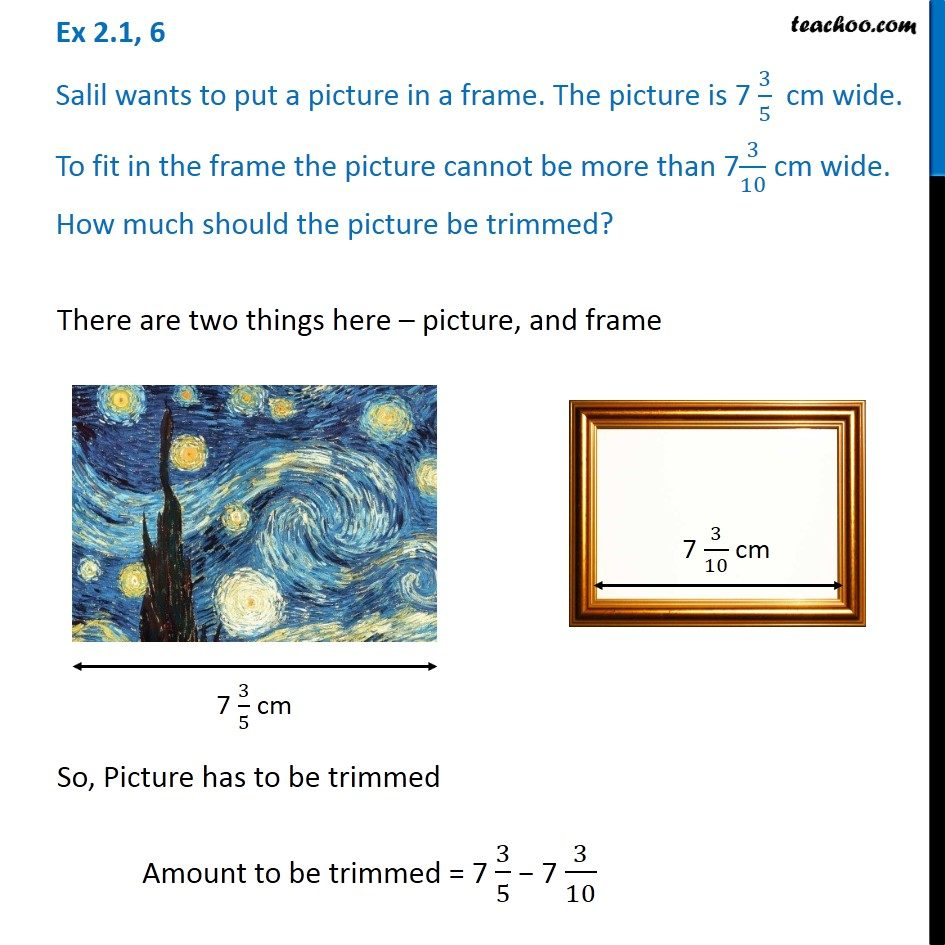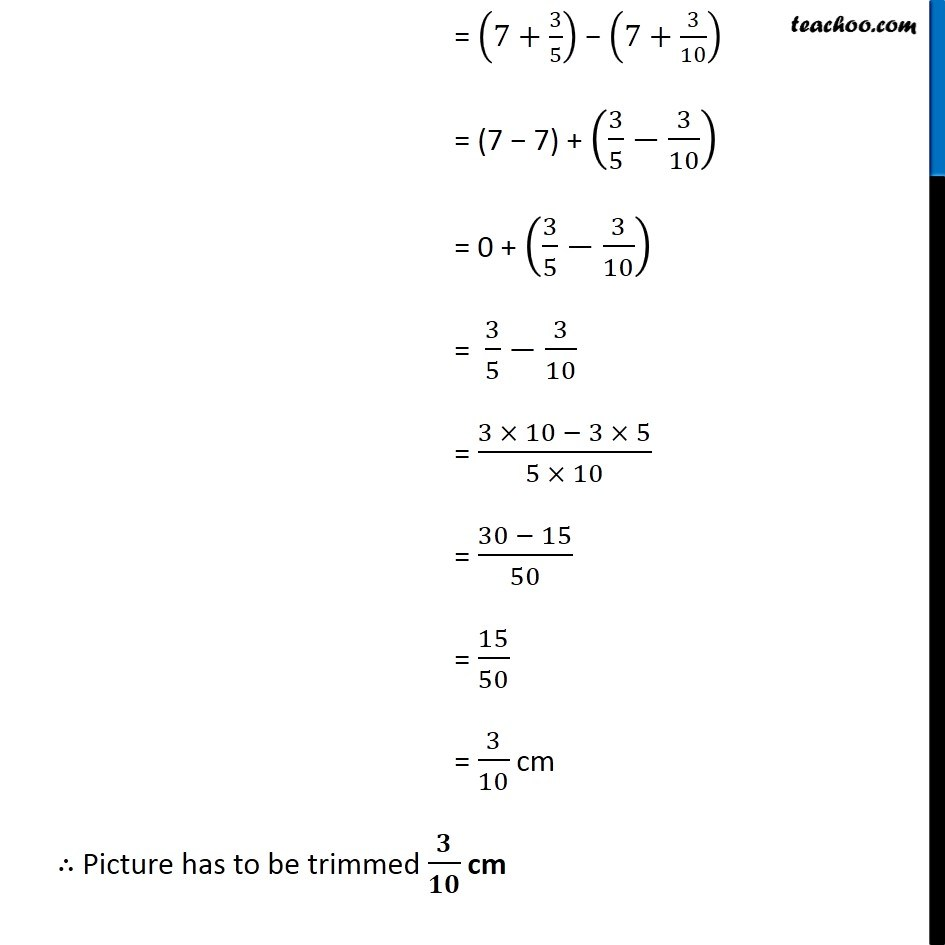Ex 2.1

Chapter 2 Class 7 Fractions and Decimals
Serial order wiseGet live Maths 1-on-1 Classs - Class 6 to 12

### Transcript

Ex 2.1, 6 Salil wants to put a picture in a frame. The picture is 7 3/5 cm wide. To fit in the frame the picture cannot be more than 73/10 cm wide. How much should the picture be trimmed?There are two things here – picture, and frame So, Picture has to be trimmed Amount to be trimmed = 7 3/5 − 7 3/10 = (7+3/5) − (7+3/10) = (7 − 7) + (3/5−3/10) = 0 + (3/5−3/10) = 3/5−3/10 = (3 × 10 − 3 × 5)/(5 × 10) = (30 − 15)/50 = 15/50 = 3/10 cm ∴ Picture has to be trimmed 𝟑/𝟏𝟎 cm